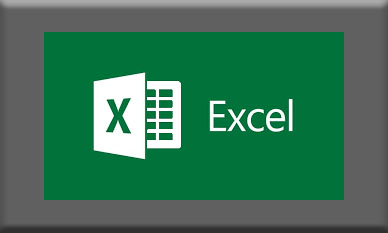home > training > 20779: Analyzing Data with Excel# 20779: Analyzing Data with Excel

\$1,895

• 3 Days
• Replay™ Class Recordings Included
Interested in scheduling a date for this course?
Register today - no risk!  No cancellation fees.  Full money back guarantee!x
Course:
• This field is for validation purposes and should be left unchanged.

Course Description

The main purpose of the course is to give students the ability to add BI techniques to Excel data analysis. The course goes beyond the capabilities of tables and charts and uses Power Query Power Pivot Tables, Pivot Charts, the Excel Data Model, and Power BI.

Outline

Module 1: Data Analysis in Excel

This module looks at the classic Excel dashboard and at ways to extend it.

Lessons

• Classic Data Analysis with Excel
• Excel Pivot Tables
• Limitations of Classic Data Analysis

Lab : Building a Classic Excel Dashboard

• Filtering and Formatting Data
• Building a Pivot Table
• Building a Pivot Chart
• Building a Dashboard

After completing this module, students will be able to:

• Describe classic data analysis with Excel
• Describe Excel pivot tables
• Describe the limitations of classic data analysis with Excel

Module 2: The Excel Data Model

This module looks at the classic Excel data model and at ways to extend it.

Lessons

• Using an Excel Data Model
• DAX

Lab : Explore an Excel Data Model

• Create Calculated Columns
• Format Data Model Data
• Create Measures
• Analyze the Data

After completing this module, students will be able to:

• Describe an Excel data model
• View data within an Excel data table
• Describe DAX

Module 3: Importing Data from Files

This module looks at pre-formatting and importing CSV files.

Lessons

• Importing Data into Excel
• Shaping and Transforming Data

Lab : Importing Data from a CSV File

• Import and Transform Data from a CSV File
• Add Data from a Folder

After completing this module, students will be able to:

• Import data into excel.
• Shape and transform data.

Module 4: Importing Data from Databases

This module looks at how to import data into Excel from a SQL Server database.

Lessons

• Available Data Sources
• Previewing, Shaping, and Transforming Data
• Table Relationships and Hierarchies

Lab : Import Data from Multiple Sources

• Import Data from SQL Server
• Import Data from a CSV File
• Create a Data Table

After completing this module, students will be able to:

• Identify available data sources.
• Preview, shape, and transform data.
• Explain table relationships and hierarchies.
• Load data from various sources.

Module 5: Importing Data from Excel Reports

This module describes how to import data from a report.

Lessons

• Importing Data from Excel Reports
• Transforming Excel report Data

Lab : Importing Data from a Report

• Import Data from Excel
• Transform the Excel Data
• Load the Data into an Excel Data Model

After completing this module, students will be able to:

• Import data from Excel reports.
• Transform Excel report data.

Module 6: Creating and Formatting Measures

This module describes how to create and format measures.

Lessons

• DAX
• Measures

Lab : Creating Measures using Advanced DAX Functions

• Last year comparison
• Year to date
• Market Share

After completing this module, students will be able to:

• Explain what DAX is and when to use it.
• Describe a measure.
• Use some of the advanced functions within DAX.

Module 7: Visualizing Data in Excel

This module describes how to visualize data in Excel.

Lessons

• Pivot Charts
• Cube Functions
• Charts for Cube Functions

Lab : Data Visualization in Excel

• Create a Tabular Report
• Create a Pivot Chart

After completing this module, students will be able to:

• Create and refine a pivot chart.
• Describe cube functions and when to use them.
• Describe a number of charts for use with cube functions.

Module 8: Using Excel with Power BI

This module describes how to use Excel with Power BI.

Lessons

• Power BI
• Power BI Mobile App

Lab : Creating a Power BI Dashboard with Excel

• Creating a Power BI Dashboard

After completing this module, students will be able to:

• Describe Power Bi and the various versions available.
• Upload Excel data to Power BI.
• Describe the Power BI App.

Audience

This course is intended for anyone responsible for analyzing data with Excel.
The course will likely be attended by SQL Server report creators who are interested in alternative methods of presenting data.

Prerequisites

Before attending this course, students must have:

• Basic knowledge of the Microsoft Windows operating system and its core functionality
• Good Knowledge of Excel spreadsheets, functions, charts and pivot tables
• Working knowledge of relational databases
What You Will Learn

After completing this course, students will be able to:

• Explore and extend a classic Excel dashboard
• Explore and extend an Excel data model
• Pre-format and import a .CSV file
• Import data from a SQL Server database
• Import data from a report
• Create measures using advanced DAX functions
• Create data visualizations in Excel
• Create a Power BI dashboard with Excel